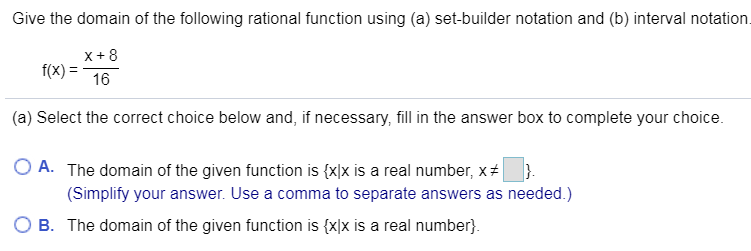# Give the domain of the following rational function using (a) set-builder notation and (b) interval notation.x+8f(x)16(a) Select the correct choice below and, if necessary, fill in the answer box to complete your choiceO A. The domain of the given function is {x]x is a real number, x #(Simplify your answer. Use a comma to separate answers as needed.)B The domain of the given function is {xlx is a real number

Question
1 viewshelp_outlineImage TranscriptioncloseGive the domain of the following rational function using (a) set-builder notation and (b) interval notation. x+8 f(x) 16 (a) Select the correct choice below and, if necessary, fill in the answer box to complete your choice O A. The domain of the given function is {x]x is a real number, x # (Simplify your answer. Use a comma to separate answers as needed.) B The domain of the given function is {xlx is a real number fullscreen
check_circle

Step 1

The given funct...

### Want to see the full answer?

See Solution

#### Want to see this answer and more?

Solutions are written by subject experts who are available 24/7. Questions are typically answered within 1 hour.*

See Solution
*Response times may vary by subject and question.
Tagged in

### Other